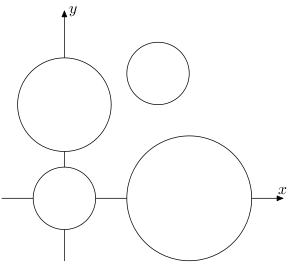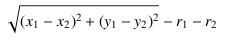# The Closest Circle

Time Limit : 8 sec, Memory Limit : 131072 KB

# The Closest Circle

You are given N non-overlapping circles in xy-plane. The radius of each circle varies, but the radius of the largest circle is not double longer than that of the smallest.Figure 1: The Sample Input

The distance between two circles C1 and C2 is given by the usual formulawhere (xi, yi ) is the coordinates of the center of the circle Ci, and ri is the radius of Ci, for i = 1, 2.

Your task is to write a program that finds the closest pair of circles and print their distance.

## Input

The input consists of a series of test cases, followed by a single line only containing a single zero, which indicates the end of input.

Each test case begins with a line containing an integer N (2 ≤ N ≤ 100000), which indicates the number of circles in the test case. N lines describing the circles follow. Each of the N lines has three decimal numbers R, X, and Y. R represents the radius of the circle. X and Y represent the x- and y-coordinates of the center of the circle, respectively.

## Output

For each test case, print the distance between the closest circles. You may print any number of digits after the decimal point, but the error must not exceed 0.00001.

```4
1.0 0.0 0.0
1.5 0.0 3.0
2.0 4.0 0.0
1.0 3.0 4.0
0
```

## Output for the Sample Input

```0.5
```

Source: ACM-ICPC Japan Alumni Group Summer Camp 2006 , Day 1, Tokyo, Japan, 2006-09-22
http://jag-icpc.org/